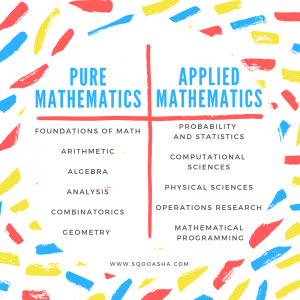# The Many Kinds of Math

Some of you may have wondered at one point or another this question: how many different kinds of math are there? Almost everyone that has reached adulthood has heard of algebra, calculus, geometry, probability and statistics, but just how many different areas of mathematics exists is the question.

Mathematics encompasses a growing variety of subjects and some of these subjects are difficult to pin down to just one category of classification. That said, there are two distinct divisions of mathematics: pure mathematics and applied mathematics. The difference is that pure mathematics is studied for its fundamental concentration and applied mathematics is studied for its real-world uses.

The many kinds of math are split up between pure and applied mathematics.This list, however, is not all-encompassing. There are many subsections to each of these broad subjects. The list above is simply to give you an idea of just how many subjects there are, and how they are organized. Like the history of math itself, these lists and categories will change over time as newly created subjects arise and newly discovered links between areas occur. Math is ever-changing! Your can read more about this topic at Lists of Mathematics Topics.